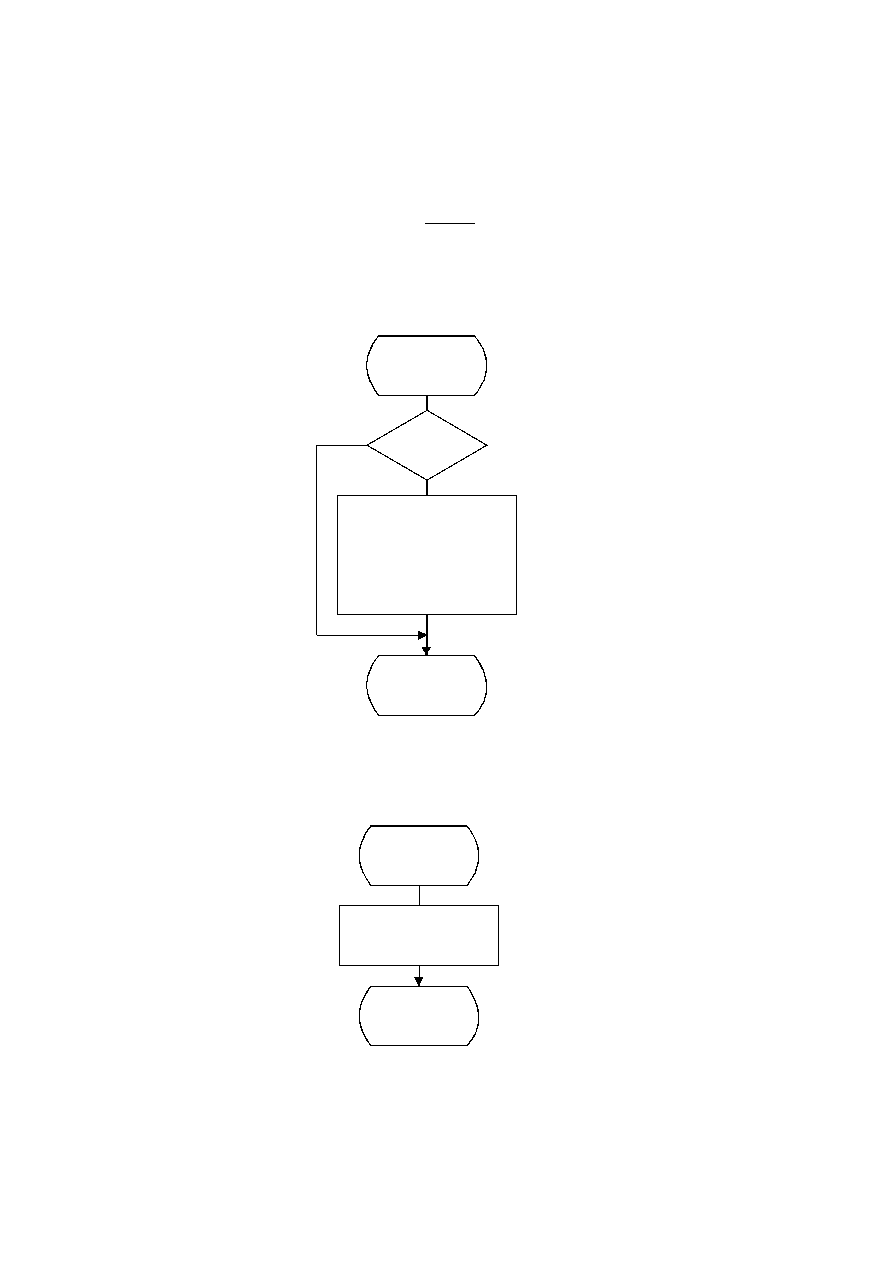ISO/IEC 10918-1 : 1993(E)
In Figure G.3, Ss is the start of spectral selection, Se is the end of spectral selection, K is the index into the list of
coefficients stored in the zig-zag sequence ZZ, R is the run length of zero coefficients, and EOBRUN is the run length of
EOBs. EOBRUN is set to zero at the start of each restart interval.
If the scan header parameter Al (successive approximation bit position low) is not zero, the DCT coefficient values ZZ(K)
in Figure G.3 and figures which follow in this annex, including those in the arithmetic coding section, shall be replaced
by the point transformed values ZZ'(K), where ZZ'(K) is defined by:
ZZ'(K) =
ZZ(K)x
2
Al
EOBSIZE is a procedure which returns the size of the EOB extension field given the EOB run length as input. CSIZE is a
procedure which maps an AC coefficient to the SSSS value defined in the subclauses on sequential encoding (see F.1.1
and F.1.3).
TISO1600-93/d098
Encode_EOBRUN
EOBRUN = 0
?
Yes
No
SSSS = EOBSIZE(EOBRUN)
I = SSSS × 16
Append EHUFSI(I)
bits of EHUFCO(I)
Append SSSS low order
bits of EOBRUN
EOBRUN = 0
Done
Figure G.4 ­ Progressive encoding of a non-zero AC coefficient
Figure G.4  = 11 cm = 430 %
TISO1610-93/d099
Encode_ZRL
Append EHUFSI(X'F0')
bits of EHUFCO(X'F0')
R = R ­ 16
Done
Figure G.5 ­ Encoding of the run of zero coefficients
124
CCITT Rec. T.81 (1992 E)